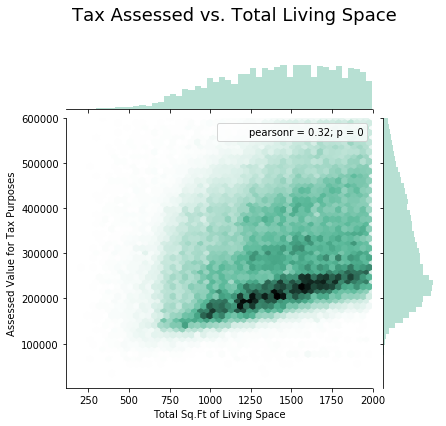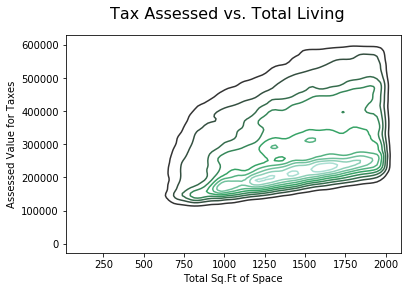# Exploration with Hexagonal Binning and Contour Plots

• Last Updated : 21 Apr, 2022

Hexagonal binning is a plot of two numeric variables with the records binned into hexagons. The code below is a hexagon binning plot of the relationship between the finished square feet versus the tax-assessed value for homes. Rather than plotting points, records are grouped into hexagonal bins and color indicating the number of records in that bin. To get the csv file used, click here. Loading Libraries

## Python3

 `import` `numpy as np``import` `pandas as pd``import` `seaborn as sns``import` `matplotlib.pyplot as plt`

## Python3

 `data ``=` `pd.read_csv("kc_tax.csv")` `print` `(data.head())`

Output:

```   TaxAssessedValue  SqFtTotLiving  ZipCode
0               NaN           1730  98117.0
1          206000.0           1870  98002.0
2          303000.0           1530  98166.0
3          361000.0           2000  98108.0
4          459000.0           3150  98108.0```

Data info

## Python3

 `print` `(data.shape)``print` `("\n", data.info())`

Output:

```(498249, 3)

RangeIndex: 498249 entries, 0 to 498248
Data columns (total 3 columns):
TaxAssessedValue    497511 non-null float64
SqFtTotLiving       498249 non-null int64
ZipCode             467900 non-null float64
dtypes: float64(2), int64(1)
memory usage: 11.4 MB```

Selecting data

## Python3

 `# Take a subset of the King County, Washington``# Tax data, for Assessed Value for Tax purposes``# < \$600, 000 and Total Living Sq. Feet > 100 &``# < 2000` `data ``=` `data.loc[(data[``'TaxAssessedValue'``] < ``600000``) &``                ``(data[``'SqFtTotLiving'``] > ``100``) &``                ``(data[``'SqFtTotLiving'``] < ``2000``)]`

Checking for null-value

## Python3

 `# As you can see in the info``# that records are not complete``data[``'TaxAssessedValue'``].isnull().values.``any``()`

Output:

`False`

Code #1: Hexagonal Binning

## Python3

 `x ``=` `data[``'SqFtTotLiving'``]``y ``=` `data[``'TaxAssessedValue'``]` `fig ``=` `sns.jointplot(x, y, kind ``=``"``hex``",``                    ``color ``=``"``# 4CB391")` `fig.fig.subplots_adjust(top ``=` `0.85``)` `fig.set_axis_labels(``'Total Sq.Ft of Living Space'``,``                    ``'Assessed Value for Tax Purposes'``)` `fig.fig.suptitle(``'Tax Assessed vs. Total Living Space'``,``                 ``size ``=` `18``);`

Output:Contour Plot : A contour plot is a curve along which the function of two variable, has a constant value. It is a plane section of the three-dimensional graph of the function f(x, y) parallel to the x, y plane. A contour line joins points of equal elevation (height) above a given level. A contour map is a map is illustrated in the code below. The contour interval of a contour map is the difference in elevation between successive contour lines. Code #2: Contour Plot

## Python3

 `fig2 ``=` `sns.kdeplot(x, y, legend ``=` `True``)` `plt.xlabel(``'Total Sq.Ft of Space'``)` `plt.ylabel(``'Assessed Value for Taxes'``)` `fig2.figure.suptitle(``'Tax Assessed vs. Total Living'``, size ``=` `16``);`

Output:My Personal Notes arrow_drop_up